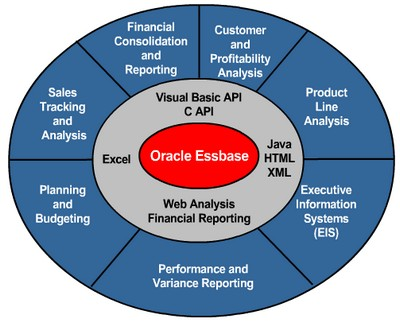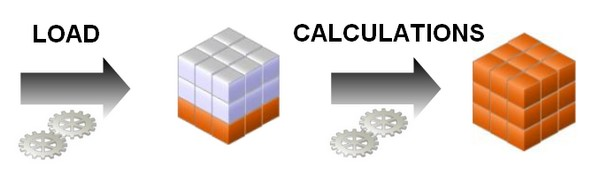# Essbase - Consolidation Operator

The consolidations operator define the consolidation path and can be used in formulas.

It defines how Essbase rolls up data for each member in a branch to the parent.

## List of operator

Operator Representation Description
+ plus sign representing addition The default operator. Essbase adds the member to the result of previous calculations performed on members of the branch.
dash representing subtraction Essbase multiplies the member by ‑1 and then adds the product to the result of previous calculations performed on members of the branch.
* asterisk representing multiplication Essbase multiplies the member by the result of previous calculations performed on members of the branch.
/ forward slash representing division Essbase divides the member into the result of previous calculations performed on members of the branch.
% percent sign representing percentage Essbase divides the member into the sum of previous calculations performed on members of the branch. The result is multiplied by 100.
~ tilda representing no consolidation Does not use the member in the consolidation to its parent.
^ Does not use the member in any consolidation in any dimension.

## Example

Consolidation operators define how Essbase rolls up data for each member in a branch to the parent.

For example, using the default addition (+) operator, Essbase adds 100‑10, 100‑20, and 100‑30 and stores the result in their parent, 100, as shown below in the TBC Product Dimension.

The Product dimension contains mostly addition (+) operators, which indicate that each group of members is added and rolled up to the parent.

Diet has a tilde (~) operator, which indicates that Essbase does not include the Diet member in the consolidation to the parent, Product. The Diet member consists entirely of members that are shared. The TBC product management group wants to be able to isolate Diet drinks in reports, so TBC created a separate Diet member that does not impact overall consolidation.

## Precedence

Operator Precedence: Calculating Members with Different Operators (Effect of Position)

Essbase calculates the data of a branch in top-down order. For example, if you have, in order, two members tagged with an addition (+) operator and a third member tagged with a multiplication (*) operator, Essbase adds the first two and multiplies that sum by the third.

Because Essbase always begins with the top member when it consolidates, the order and the labels of the members is important.

When siblings have different operators, Essbase calculates the data in top-down order. The following section describes how Essbase calculates the members listed below:

``````Parent1
Member1 (+)   10
Member2 (+)   20
Member3 (-)   25
Member4 (*)   40
Member5 (%)   50
Member6 (/)   60
Member7 (~)   70
```
```

Essbase calculates Member1 through Member4 as follows:

``````(((Member1 + Member2) + (-1)Member3) * Member4) = X
(((10 + 20) + (-25)) * 40) = 200```
```

If the result of this calculation is X, Member5 consolidates as follows:

``````(X/Member5) * 100 = Y
(200/50) * 100 = 400```
```

If the result of the Member1 through Member4 calculation is Y, Member6 consolidates as follows:

``````Y/Member6 = Z
400/60 = 66.67```
```

Because Member7 is set to No Consolidation(~), Essbase ignores Member7 in the consolidation.

Discover MoreEssbase - (Consolidations|Aggregations)

Member consolidation Operators, determine how children roll up into their parents. Consolidation define how the data are aggregate. Consolidations are also known as aggregation rules. Consolidation...Essbase - Calculations

Essbase includes powerful calculation features for demanding analytic requirements. A rich library of functions makes it easy to define advanced and sophisticated business logic and relationships. Essbase...Essbase - Consolidation Path

The definition of the consolidation operator define the consolidation path. The TBC application has several consolidation paths: Individual products roll up to product families, and product families...Essbase - Formulas

Formulas calculate relationships between member in the database outline. You can : apply formulas to members in the outline, or place formulas in a calculation script. This topic explains how TBC...Essbase - Outline-based Calculations

Outline Calculations represent the simplest method of calculations. In block storage outline calculations, Essbase calculates the essbase database according to : the relationship between member in the...Essbase - The OLAP Design Cycle (to create an optimized database)

A contains two types of values : the values that you enter or load (input data) and the values that are calculated from the input data (calculated data) Then a typical conforms to the following...